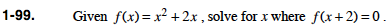### Home > PC > Chapter 1 > Lesson 1.3.1 > Problem1-99

1-99.

Given f(x) = x2 + 2x, solve for x where f(x + 2) = 0. Homework Help ✎Substitute (x + 2) for every x in the f(x) function.

Remember the middle term when you expand (x + 2)2.